Finding Numerical Derivatives

While the TI-84+ calculators are not symbolic manipulators (they cannot tell you the algebraic expression for a derivative), they can tell you an approximate numerical value for a derivative at a specific location.  Several methods may be used.

 Method 1: using nDeriv(expression, variable, value, [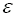])

The nDeriv command uses the symmetric difference quotient method, which approximates the numerical derivative value as the slope of the secant line,  f ' (x) = ( f (x +) - f (x -)) / 2.  Asbecomes smaller, the answer becomes more accurate.
Be careful!!!   The use of this method can yield an incorrect answer at non-differentiable points.
The default value foris 1 E -3.

EXAMPLE: Find the numerical value of the derivative for the function stated below at the indicated location (express answer to three decimal places).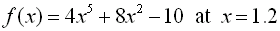Step 1:Enter the function into Y= Step 2: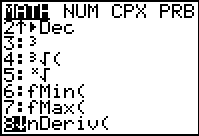From the HOME screen, hit the  MATH key and arrow down to choice #8 nDeriv(. Step 3: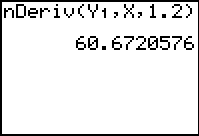The parameters for nDeriv are expression, variable, value.  (A fourth parameter exists involving the size of epsilon and is usually left off.) Hit ENTER .for the answer.

 Method 2: using thefeature and the graph

Let's examine that same problem again:

Find the numerical value of the derivative for the function stated below at the indicated location (express answer to three decimal places).Step 1:Enter the equation into Y= Step 2: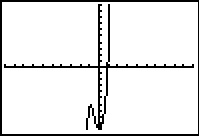Hit GRAPH.  Be sure that the WINDOW includes the x value that you will be investigating.  If not, adjust the WINDOW. Step 3:Hit the MATH key and choose #6 dy/dx.  Hit ENTER. Step 4: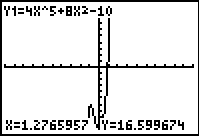The graph will reappear. (If more than one graph appears, use the up/down arrows to select the desired graph.) Use the left ◄ and right ►arrows to locate the point you wish to investigate, or simply type the point. Hit ENTER. Step 5: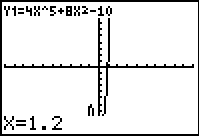Simply type the desired value on the keypad.  The number will appear below the graph. Step 6: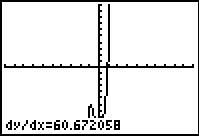Hitting ENTER will reveal the answer.